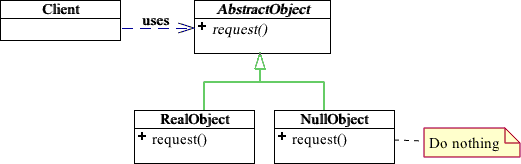# Null object pattern

Many times functions accept object references that can be null, and we tend to add if statements to treat the special case where null is passed to a function, and either provide a default response or do nothing. In the following example our calculateSpeed function expects an object that has a getSpeed function. In Javascript we will have to do something like:

``````class Car {
getSpeed(){
return 100;
}
}

const calculateSpeed = function(vehicle){
if(vehicle && typeof vehicle.getSpeed === 'function'){
return vehicle.getSpeed();
} else {
return 50;
}
}

const car1 = new Car();

console.log(calculateSpeed(car1)); // 100
console.log(calculateSpeed()); // 50``````

But there is a better way to achieve that. Using the Null Object Pattern we can create a class that acts as vehicle, lets call it `DefaultMovable`.

``````class DefaultMovable {
getSpeed(){
return 50;
}
}``````

Our `DefaultMovable` class provides the default functionality (aka the else in our previous code snippet), that way we can avoid the if/else statement.

``````class Car {
getSpeed(){
return 100;
}
}

class DefaultMovable {
getSpeed(){
return 50;
}
}

const calculateSpeed = function(vehicle = new DefaultMovable()){
return vehicle.getSpeed();
}

const car1 = new Car();

console.log(calculateSpeed(car1)); // 100
console.log(calculateSpeed()); // 50``````

The UML diagram of this pattern will look like this:The same example in Ruby would look like:

``````class Car
def get_speed
100
end
end

class DefaultMovable
def get_speed
50
end
end

def getSpeed(vehicle = DefaultMovable.new)
vehicle.get_speed();
end``````

This is just a pattern and as every pattern it has its pros and cons, apply it thoughtfully based on your use case. (The example is fictional for the shake of demonstrating the pattern)

Published 9 Aug 2018